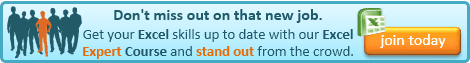## Tuesday, 15 July 2014

### Excel Tip: Find a code in among other text

I've got a little bit of news before we get into this week's post.

Regular readers of the blog will know that I am a big fan of Mynda Treacy's Excel Dashboards course. Well, Mynda is making it available once again, for a limited period. You can get it here - and if you sign up by the 24th July, you will not only get a 20% discount, but I will give you a copy of my Introduction to Excel Pivot Tables video course, absolutely free.

So, if you've missed out before, click over there now, before you miss out again.

OK. On with this week's post.

Have you ever had a problem where account codes, or suchlike, are embedded in larger sections of text, when you need them in a field of their own (for lookups or pivot tables for example)?

Well, here's a little trick that will work in certain circumstances.

I had an issue yesterday with a client who needed to analyse transactions by project code, however the project code did not have it's own field in the system and was entered, along with a number of other pieces of information in the description field. What's more, the code could appear anywhere within the description field.

The key to making this work, is being able to identify something within the code that will not appear elsewhere in the text. In my client's example, all project codes started with PROJ.

We can use the FIND function for this:

=FIND(find_text,within_text,[start_num])

The FIND function returns the position of find_text within the larger text, within_text. The optional argument, start_num, allows you to specify at what position to start looking (if this is argument is not entered, the FIND function will start at the beginning of within_text).

Say the cell A1 contains the following text:

"Expenses for PROJ13245 but that is not the only text here"

the function

=FIND("PROJ",A1)

will return 14, the position in the text of the first letter of PROJ.

If the project code is always 9 digits, then we can use the MID function with FIND to pull it out.

The MID function is structured thus:

=MID(text,start_num,num_chars)

This returns the num_chars of text from text, starting at start_num, so:

=MID(A1,14,9)

would return our project code, if we already knew that it started at position 14, however we can replace the 14 with our FIND function, to calculate the start of the Project Code, so:

=MID(A1,FIND("PROJ",A1),9)

will pull out the 9 digit project code from the text.

But what if the length of the code was variable, well, if we can assume that it will always be followed by a space, for example, we can use find again to identify the end, and this calculate num_chars.

We can find the position of the space at the end of the project code, by using the following FIND function:

=FIND(" ",A1,FIND("PROJ",A1))

All we have done is used our earlier calculation of the start of the project code, FIND("PROJ",A1), as the start_num value in a new FIND looking for the space. This will return the position of the first space following the letters PROJ.

To calculate the num_chars we need for our MID function, we just need to deduct the start position, i.e. FIND("PROJ",A1). So,

=FIND(" ",A1,FIND("PROJ",A1))-FIND("PROJ",A1)

will return the length of the project code to replace the 9 in our MID function:

=MID(A1,FIND("PROJ",A1),FIND(" ",A1,FIND("PROJ",A1))-FIND("PROJ",A1))

which will return the project code, PROJ13245.

And that's it. It obviously won't work in every situation, but there are plenty where it, or a version of it, will.

Good luck!If you enjoyed this post, go to the top of the blog, where you can subscribe for regular updates and get two freebies "The 5 Excel features that you NEED to know" and "30 Chants for Better Charts".

#### 4 comments:

1.nice - but is the find function upper/lower case specific? how can you avoid that?

1.It is case specific. To stop it being case specific, you could just replace each reference to A1 in the formula with UPPER(A1), thereby converting all of A1 to upper case before using FIND.

2.Fine, but can the "within_text" be a range, such as A1:C1 or A1:C5?

1.The reason for doing it would normally be because you need to apply it to multiple cells. In your first example you could enter the formula above in cell A2 and copy along the row to C2, stripping the code out of each of the cells above. Similarly for your second example, you could enter the formula into cell D1 and copy it across and down to cover the range D1:F5.